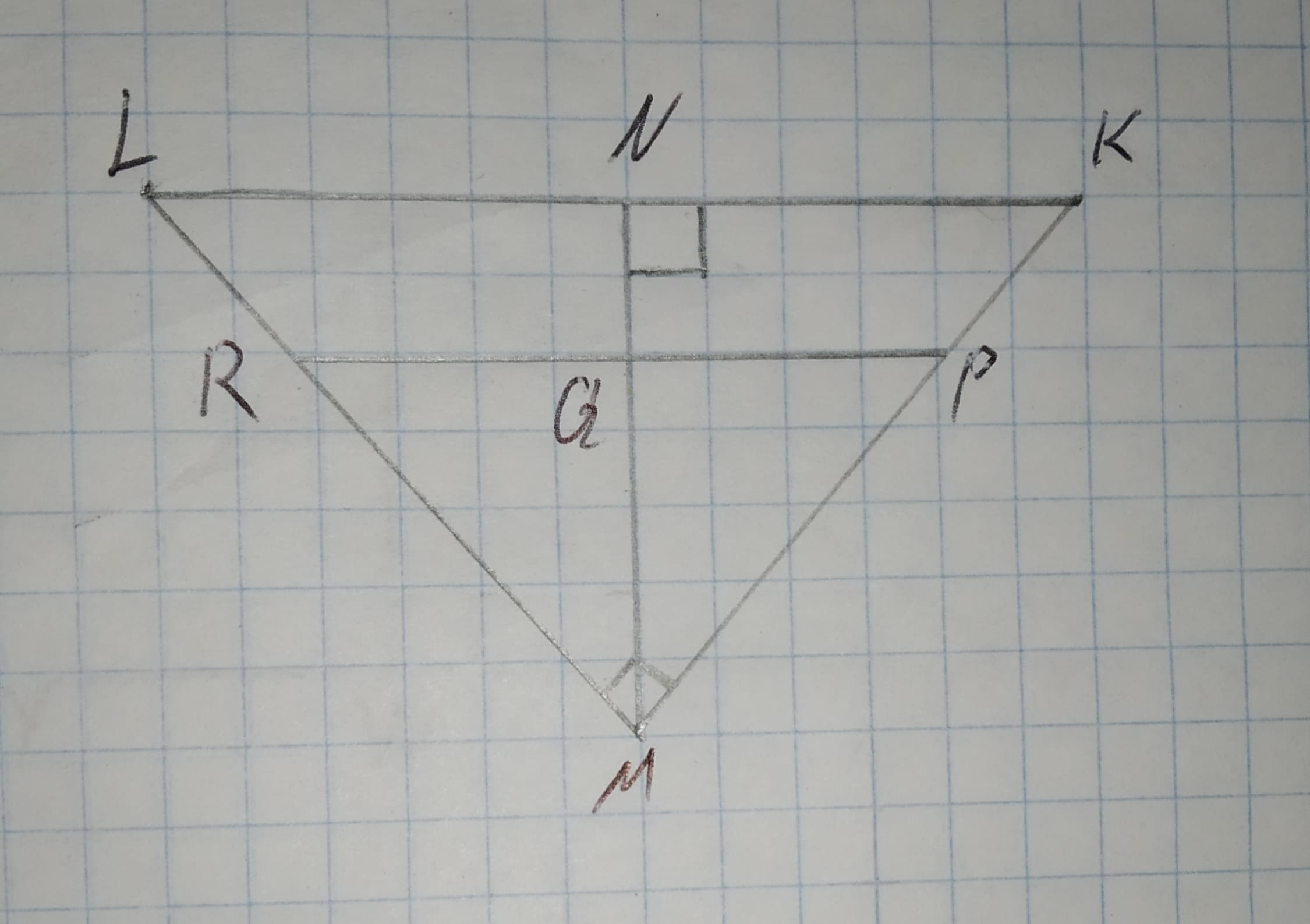To find: The values of KP, KM, MR, ML, MN and PR.Given:12210203131.jpgbar(PR)||bar(KL) = 9, ln = 16, PM = 2(KP)KM=KP+PM=3KPChaya Galloway 2021-01-10 Answered

To find: The values of KP, KM, MR, ML, MN and PR.
Given:$$\displaystyle\overline{{{P}{R}}}{\mid}{\mid}\overline{{{K}{L}}}={9},{\ln{=}}{16},{P}{M}={2}{\left({K}{P}\right)}$$
KM=KP+PM=3KP

• Questions are typically answered in as fast as 30 minutes

Solve your problem for the price of one coffee

• Math expert for every subject
• Pay only if we can solve itfunblogC

Conclusion:
By A-A similarity, it can be proved that:
$$\displaystyle\triangle{L}{M}{K}\sim\triangle{M}{N}{K}$$
Similar triangles have corresponding sides as proportional.
$$\displaystyle\frac{{{M}{K}}}{{{L}{K}}}=\frac{{{N}{K}}}{{{M}{K}}}$$
$$\displaystyle\Rightarrow\frac{{{3}{K}{P}}}{{25}}=\frac{{9}}{{{3}{K}{P}}}$$
$$\displaystyle\Rightarrow{K}{P}={5}$$
$$\displaystyle\Rightarrow{K}{M}={3}{K}{P}={15}$$
By A-A similarity, it can be proved that:
$$\displaystyle\triangle{L}{M}{K}\sim\triangle{R}{M}{P}$$
Similar triangles have corresponding sides as proportional.
$$\displaystyle\frac{{{M}{K}}}{{{L}{K}}}=\frac{{{P}{M}}}{{{R}{P}}}$$
$$\displaystyle\Rightarrow\frac{{15}}{{25}}=\frac{{10}}{{{R}{P}}}$$
$$\displaystyle\Rightarrow{K}{P}={16}\frac{{2}}{{3}}$$
Using Pythagorean Theorem in right angled triangle $$\displaystyle\triangle{L}{M}{K}$$:
$$\displaystyle{H}{y}{p}{o}{t}{e}nu{s}{e}^{{2}}={B}{a}{s}{e}^{{2}}+{P}{e}{r}{p}{e}{n}{d}{i}{c}ul{{a}}{r}^{{2}}$$
$$\displaystyle={25}^{{2}}={15}^{{2}}+{L}{M}^{{2}}$$
$$\displaystyle\Rightarrow{L}{M}^{{2}}={400}$$
$$\displaystyle\Rightarrow{L}{M}={20}$$
In similar triangles: $$\displaystyle\triangle{L}{M}{K}\sim\triangle{M}{N}{K}$$
Ratio can be written as:
$$\displaystyle\frac{{{L}{M}}}{{{M}{K}}}=\frac{{{M}{N}}}{{{N}{K}}}$$
$$\displaystyle\Rightarrow\frac{{20}}{{15}}=\frac{{{M}{N}}}{{9}}$$
$$\displaystyle\Rightarrow{M}{N}={12}$$
$$\displaystyle{K}{P}={5},{K}{M}={15},{M}{R}={13}\frac{{1}}{{3}},{M}{L}={20},{M}{N}={12},{P}{R}={16}\frac{{2}}{{3}}$$